### Re: Whats up with the Lewis dot structure of sulfate?

Date: Fri Jan 28 12:38:22 2000
Posted By: Dan Berger, Faculty Chemistry/Science, Bluffton College
Area of science: Chemistry
ID: 948511245.Ch
Message:

Whats up with the Lewis dot structure of sulfate?

I Would like to have the structure of "covalent coordinate" molecules explained in terms of either molecular orbitals or hybridizations. Specifically, I am interested in the sulfate ion and carbond monoxide. I am not interested in hearing terms like "filling the valence shell" or "becoming like a noble gas". Thanks

Josh, I remember you. You seem to be taking a strongly realistic view of things like orbitals and so forth, which is not entirely defensible (see, for example, Eric Scerri's "The Periodic Table and the Electron" in the Nov-Dec 1997 American Scientist). An orbital is not something we would call an "observable" in quantum mechanics. Only the total electron density is observable, and if Lewis dot structures can help you predict the total electron density they're just as good as a molecular orbital picture. In fact, it is my opinion (for which I am in the process of constructing a formal argument) that Lewis structures now function as a shorthand for the valence-bond picture of bonding.

Second, if you want the full explanation in terms of "molecular orbitals or hybridizations," you really ought to read the references given in this MadSci answer. Incidentally, at least one of those references concluded that the Lewis dot picture (with octets and formal charges on sulfur) is a better fit to the electron density distribution than the "expanded valence" picture we get from hybridization theory (for which, of course, the best source is Pauling's The Nature of the Chemical Bond).

But I'll see what I can do for you, in terms of both molecular orbital theory and valence-bond theory - qualitatively, of course. A good general reference for this sort of thing is F.A. Cotton's classic text, Chemical Applications of Group Theory. If you want the full mathematical treatment of what is presented schematically below, you should work your way through a copy. Even then you won't have the mathematical equipment to do other than guess at the orbital energies...

• Valence-bond Theory considers bonds as combinations of overlapping one-electron atomic orbitals, which may or may not be hybridized. This approach is set out in many texts but is usually represented using conventions drawn from Lewis theory, which was first proposed by G.N. Lewis in "The Atom and the Molecule," Journal of the American Chemical Society 38, 762-785 (1916).
• Molecular Orbital Theory, in its simplest form (e.g. Hückel and Extended Hückel Theory), combines atomic orbitals to form molecular orbitals according to the symmetry of the molecule, then fills them two electrons at a time. More sophisticated versions of molecular orbital theory exist, with various ways of taking electron-electron interactions into account, but these are usually add-ons to the basic method.

### 1. Carbon monoxide in terms of hybridization and resonance (i.e. valence bond) theory.

CºO, being linear, is described by hybridization theory as having sp-hybridized atoms. Carbon begins with one electron in each of the following orbitals: sp1, sp2, px, py, shown schematically in Figure 1.

Oxygen begins with six valence electrons, distributed (for example) thus: one in sp1, two in sp2, two in px, one in py, shown schematically in Figure 2.Figure 1 Figure 2

However, the one-electron orbitals on oxygen and carbon don't match up well, so we can fix things one of two ways:

1. We "move" (this is pure bookkeeping!) an electron from oxygen's px to carbon's sp1, so that both atoms now have the same electron configuration and can form a triple bond by the overlap of the one-electron orbitals (Figure 3). There is one lone pair each on carbon and on oxygen, and because we have "removed" an electron from oxygen and "given" it to carbon, there are formal charges.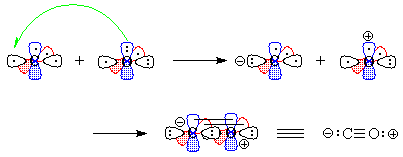Figure 3
2. We "move" an electron from px on carbon to carbon's sp1. Overlap of the remaining one-electron orbitals results in a double bond, with one lone pair of electrons on carbon and two on oxygen; all this is shown schematically in Figure 4.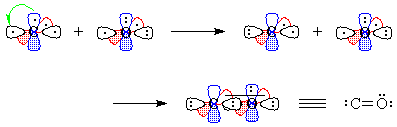Figure 4

Of course, valence-bond theory requires that we mix these two models (which can in fact be stated mathematically); this is called "resonance" and is represented schematically in Figure 5. The true structure is neither of these models, but a combination, and includes contributions from electronegativity which shift electron density from carbon back to oxygen. The calculated charge density surface (HF/6-31G*) is shown in Figure 6.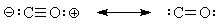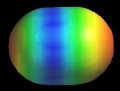Figure 5 Figure 6

### 2. Carbon monoxide in terms of molecular orbital theory.

Qualitatively this is an extremely simple picture. The valence atomic orbitals on carbon and oxygen are, to a crude first approximation, identical. Since the molecular point group is C∞ v, there are only two possible orbital symmetries: S and P. The s and pz atomic orbitals have S symmetry, while the px and py have P symmetry.

More strictly speaking, we need to realize that neither the C nor the O are at the molecule's center of symmetry, so that we must take symmetry-adapted linear combinations of the atomic orbitals -- which then turn out to be molecular orbitals right off. In point of fact, we don't usually work through the group-theoretical analysis because simple visual combination gets us to the same place. And so, to work.

There are two sets of orbitals of P symmetry, and they are mutually perpendicular so that they cannot overlap. They combine to form two pairs of degenerate molecular orbitals, one pair bonding (p) and the other antibonding (p*). These are shown in Figure 7.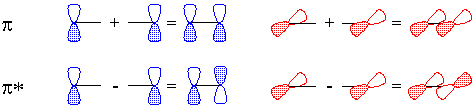Figure 7

There are also two sets of orbitals of S symmetry, and they are not mutually perpendicular. We can combine them in two equivalent ways:

1. We can first take independent combinations of the atomic s and pz orbitals, and then allow the sets to interact (Figure 8).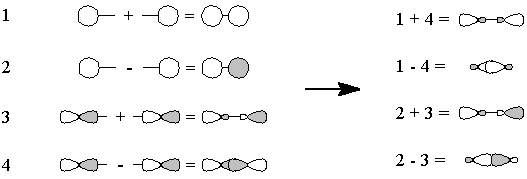Figure 8
2. We can first combine the s and pz orbitals on each atom, producing sp hybrids, and then form combinations suited to the overall molecular symmetry (Figure 9).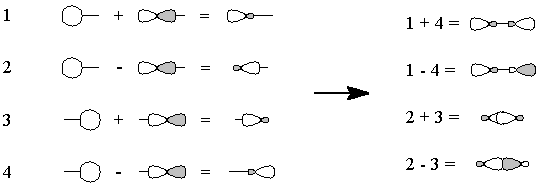Figure 9
Once we have the orbitals, all that remains is to order them according to energy. It turns out that the ordering is as shown in Figure 10 (again, results of an HF/6-31G* calculation), and we can fill the molecular orbitals two electrons at a time until we have used up all ten valence electrons. (s* and p* are antibonding, n is nonbonding, s and p are bonding molecular orbitals.)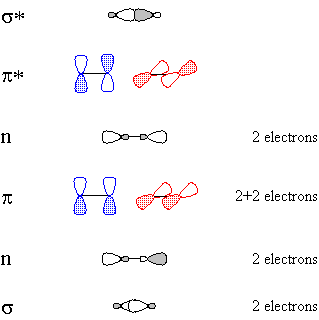Figure 10

### 3. The sulfate ion in terms of hybridization and resonance theory.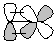Figure 11
The sulfur atom in sulfate ion is sp3 hybridized. This is dictated by the fact that it needs to form bonds to four oxygen atoms in a molecule of Td symmetry. In addition, electrons from the non-bonding p atomic orbitals on oxygen are able to form p bonds with the sulfur atom's empty d orbitals as shown in Figure 11. These p bonds are "interchanged" by resonance, and the net result is similar to that shown in the resonating Lewis structures of Figure 12.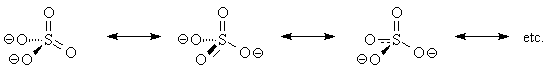Figure 12

However, this is not the only possible valence-bond picture! We can entirely ignore p-d p-bonding (which is not supported by quantum chemical calculations anyhow) and present a picture which fully satisfies the Lewis octet rule. In this representation, sulfur is still sp3 hybridized, but d-orbitals on sulfur do not participate in bonding. Instead, bonding is semipolar (that is, a combination of covalent and ionic) and can be represented by the Lewis structure of Figure 13. Of course, the +2 formal charge on sulfur is not quite a true representation. An HF/6-31G* calculation calculated the charge on sulfur to be +1.77! Induction, driven by the large separation of charge in Figure 13, pulls electrons back toward sulfur so that the charge density surface for sulfate ion (Figure 14) shows a moderation of the negative charges on the oxygen atoms (the blue color is not positive charge, just "less negative").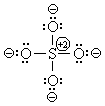Figure 13 Figure 14

### 4. The sulfate ion in terms of molecular orbital theory.

If one wants to be a purist, one can form the bonding framework of the sulfate ion exclusively from s and p orbitals on sulfur, interacting with a tetrahedral array of p orbitals on the oxygens. But it is just as valid to use Pauling's set of sp3 hybrid orbitals as a basis, as long as one combines them into sets which are compatible with the tetrahedral symmetry of the sulfate ion. These sets would have the same overall symmetry as the original atomic s, px, py and pz orbitals, and combine with appropriate combinations of the p atomic orbitals on oxygen (that is, the p orbitals which point toward sulfur).

Interestingly, sulfur d atomic orbitals (except possibly dz2) do not appear to have the proper symmetry to form molecular orbitals in a tetrahedral molecule. The additional bonds generated by molecular orbital calculations are p bonds between the oxygen atoms, in which sulfur participates very little, if at all (for an example, see Figure 15). There are also several oxygen lone-pair orbitals such as Figure 16.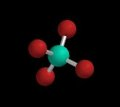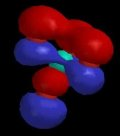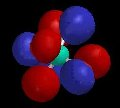sulfate Figure 15 Figure 16

Molecular orbital theory bears out the simple Lewis picture shown above in Figure 13; this is also the conclusion of A. Reed and P.v.R. Schleyer, Journal of the American Chemical Society 109, 7362-73 (1987), ibid. 112, 1434-45 (1990); E. Magnusson, ibid. 7940-51.

### 5. The bottom line

Don't knock Lewis structures too much. They tell you almost as much, at considerably less expense in time, computing power and student frustration, as the more sophisticated and "correct" valence-bond and molecular-orbital models.

The operative question, as always, is: does it correctly predict the observed electron density and chemical reactivity? For more on this, see "The Chemical Core of Chemistry" by Joachim Schummer, HYLE, 4, 129-162 (1998).

God never saw an orbital.
-- Walter Kauzmann to Maitland Jones
quoted on p. 67 of M. Jones, Organic Chemistry, Norton, 1997.

Current Queue | Current Queue for Chemistry | Chemistry archives